# （转）以 .NET 创建 Code 39 条码图片 供水晶报表打印

-------------------------------------------------

http://files.cnblogs.com/WizardWu/100914.zip

---------------------------------------------------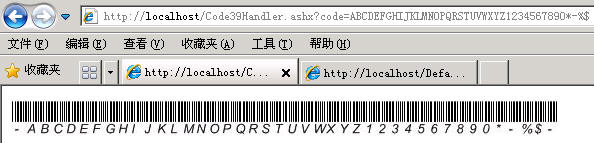<% @ WebHandler Language = " C# "  Class = " Code39Handler "   %>

using  System;
using  System.Web;
using  System.Drawing;
using  System.Drawing.Imaging;
using  System.Drawing.Text;

///   <summary>
///  用 .NET 繪圖 API，搭配條碼最普遍的 Code 39 編碼規則 (一般超商的讀條碼機都可讀)，產生條碼圖檔
///   </summary>
public   class  Code39Handler : IHttpHandler {

public   void  ProcessRequest (HttpContext context) {

// context.Response.ContentType = "text/plain";

// context.Response.Write("Hello World");

// Logic to retrieve the image file

// context.Response.ContentType = "image/jpeg";

// context.Response.WriteFile("MyImage01.jpg");

string  mycode  =  context.Request[ " code " ];

string  字串;

string  字元;

// 字串 = "*-%$*" 字串 = " * " + mycode + " * " ; // Code 39 的特性是前、後置碼會標識「星號(*)」，表示開始和結束 int 畫布高 = 35 ; int 畫布寬 = 0 ; int 筆x = 0 ; int 筆y = 20 ; // int 筆寬 = 0; if ( ! string .IsNullOrEmpty(mycode)) { 畫布寬 = 字串.Length * 13 ; Bitmap BMP = new Bitmap(畫布寬, 畫布高, System.Drawing.Imaging.PixelFormat.Format32bppPArgb); Graphics G = Graphics.FromImage(BMP); G.TextRenderingHint = TextRenderingHint.AntiAlias; G.Clear(Color.White); Brush 筆刷1 = new SolidBrush(Color.White); G.SmoothingMode = System.Drawing.Drawing2D.SmoothingMode.HighQuality; G.FillRectangle(筆刷1, 0 0 , 畫布寬, 畫布高); for ( int i = 0 ; i < 字串.Length; i ++ ) { // 取得 Code 39 碼的規則 字元 = this .genBarcode(字串.Substring(i, 1 ).ToUpper()); for ( int j = 0 ; j < 4 ; j ++ ) { if (字元.Substring(j, 1 ).Equals( " 0 " )) { G.DrawLine(Pens.Black, 筆x, 0 , 筆x, 筆y); } else { G.DrawLine(Pens.Black, 筆x, 0 , 筆x, 筆y); G.DrawLine(Pens.Black, 筆x + 1 0 , 筆x + 1 , 筆y); 筆x += 1 ; } 筆x += 1 ; if (字元.Substring(j + 5 1 ).Equals( " 0 " )) { G.DrawLine(Pens.White, 筆x, 0 , 筆x, 筆y); } else { G.DrawLine(Pens.White, 筆x, 0 , 筆x, 筆y); G.DrawLine(Pens.White, 筆x + 1 0 , 筆x + 1 , 筆y); 筆x += 1 ; } 筆x += 1 ; } // end of loop if (字元.Substring( 4 1 ).Equals( " 0 " )) { G.DrawLine(Pens.Black, 筆x, 0 , 筆x, 筆y); } else { G.DrawLine(Pens.Black, 筆x, 0 , 筆x, 筆y); G.DrawLine(Pens.Black, 筆x + 1 0 , 筆x + 1 , 筆y); 筆x += 1 ; } 筆x += 2 ; } // end of loop int x = 0 ; int addx = 13 ; G.DrawString( " - " new Font( " Arial " 10 , FontStyle.Italic), SystemBrushes.WindowText, new PointF(x, 20 )); x += addx; for ( int k = 0 ; k < mycode.Length; k ++ ) { G.DrawString(mycode.Substring(k, 1 ), new Font( " Arial " 10 , FontStyle.Italic), SystemBrushes.WindowText, new PointF(x, 20 )); x = x + addx; } G.DrawString( " - " new Font( " Arial " 10 , FontStyle.Italic), SystemBrushes.WindowText, new PointF(x, 20 )); BMP.Save(context.Response.OutputStream, ImageFormat.Jpeg); G.Dispose(); BMP.Dispose(); } else { 畫布寬 = 100 ; Bitmap BMP = new Bitmap(畫布寬, 畫布高, System.Drawing.Imaging.PixelFormat.Format32bppPArgb); Graphics G = Graphics.FromImage(BMP); G.TextRenderingHint = TextRenderingHint.AntiAlias; G.Clear(Color.White); // 未給參數時顯示的提示內容 G.DrawString( " 無條碼產生 " new Font( " 宋体 " 12 , FontStyle.Regular), SystemBrushes.WindowText, new PointF( 0 20 )); BMP.Save(context.Response.OutputStream, ImageFormat.Jpeg); G.Dispose(); BMP.Dispose(); } } // 規則可參考網址 1： http://blog.csdn.net/xuzhongxuan/archive/2008/05/28/2489358.aspx // 規則可參考網址 2： http://blog.163.com/zryou/blog/static/6903184200971704226450/ /// <summary> /// Code 39 碼的規則。 /// Code 39 碼可使用的字元如下：0~9、A~Z、+、-、*、/、%、$、. 及空白字元。

///   </summary>

///   <param name="code"></param>

///   <returns></returns>
public   string  genBarcode( string  code)
{

switch  (code)
{

case   " 0 " :
code
=   " 001100100 " ;

break ;

case   " 1 " :
code
=   " 100010100 " ;

break ;

case   " 2 " :
code
=   " 010010100 " ;

break ;

case   " 3 " :
code
=   " 110000100 " ;

break ;

case   " 4 " :
code
=   " 001010100 " ;

break ;

case   " 5 " :
code
=   " 101000100 " ;

break ;

case   " 6 " :
code
=   " 011000100 " ;

break ;

case   " 7 " :
code
=   " 000110100 " ;

break ;

case   " 8 " :
code
=   " 100100100 " ;

break ;

case   " 9 " :
code
=   " 010100100 " ;

break ;

case   " A " :
code
=   " 100010010 " ;

break ;

case   " B " :
code
=   " 010010010 " ;

break ;

case   " C " :
code
=   " 110000010 " ;

break ;

case   " D " :
code
=   " 001010010 " ;

break ;

case   " E " :
code
=   " 101000010 " ;

break ;

case   " F " :
code
=   " 011000010 " ;

break ;

case   " G " :
code
=   " 000110010 " ;

break ;

case   " H " :
code
=   " 100100010 " ;

break ;

case   " I " :
code
=   " 010100010 " ;

break ;

case   " J " :
code
=   " 001100010 " ;

break ;

case   " K " :
code
=   " 100010001 " ;

break ;

case   " L " :
code
=   " 010010001 " ;

break ;

case   " M " :
code
=   " 110000001 " ;

break ;

case   " N " :
code
=   " 001010001 " ;

break ;

case   " O " :
code
=   " 101000001 " ;

break ;

case   " P " :
code
=   " 011000001 " ;

break ;

case   " Q " :
code
=   " 000110001 " ;

break ;

case   " R " :
code
=   " 100100001 " ;

break ;

case   " S " :
code
=   " 010100001 " ;

break ;

case   " T " :
code
=   " 001100001 " ;

break ;

case   " U " :
code
=   " 100011000 " ;

break ;

case   " V " :
code
=   " 010011000 " ;

break ;

case   " W " :
code
=   " 110001000 " ;

break ;

case   " X " :
code
=   " 001011000 " ;

break ;

case   " Y " :
code
=   " 101001000 " ;

break ;

case   " Z " :
code
=   " 011001000 " ;

break ;

case   " * " :
code
=   " 001101000 " ;

break ;

case   " - " :
code
=   " 000111000 " // 好像辨識不出來
break ;

case   " % " :
code
=   " 100101000 " // 好像辨識不出來
break ;

case   " $" : code = " 010101000 " // 好像辨識不出來 break ; default : code = " 010101000 " // 都不是就印$
break ;
}

return  code;
}

public   bool  IsReusable {

get  {

return   false ;
}
}

}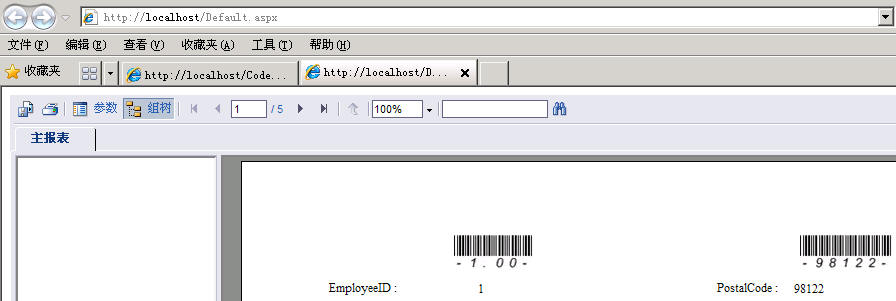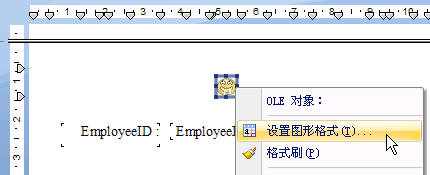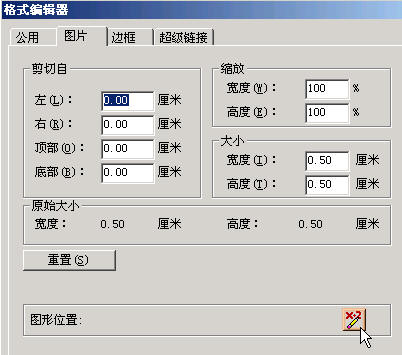"http://" & {?param1} & "/Code39Handler.ashx?code=" & {Employees.EmployeeID}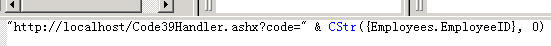C:\Inetpub\wwwroot\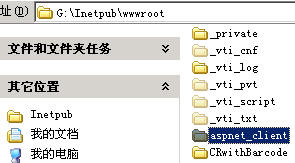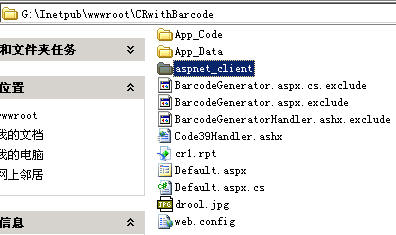09-1435
09-146509-0217
03-051万+
06-232226
05-211万+
11-162万+
11-283万+